International
Tables for
Crystallography
Volume D
Physical properties of crystals
Edited by A. Authier

International Tables for Crystallography (2006). Vol. D, ch. 1.4, pp. 101-103

## Section 1.4.3. Experimental methods

H. Küppersa*

aInstitut für Geowissenshaften, Universität Kiel, Olshausenstrasse 40, D-24098 Kiel, Germany
Correspondence e-mail: kueppers@min.uni-kiel.de

### 1.4.3. Experimental methods

| top | pdf |

#### 1.4.3.1. General remarks

| top | pdf |

Although the strain tensorand the thermal expansion tensorin general contain components with(shear strains), in practice only longitudinal effects, i.e. relative length changeswith temperature changes ΔT, are measured along different directions and the results are later transformed to a common coordinate system. Diffraction methods directly yield this ratio. Other measuring techniques require separate measurements of Δl and l. The error in the measurement of l can usually be neglected. Thus, the accuracies of Δl and ΔT limit the accuracy of thermal expansion coefficients. The temperature interval ΔT is determined by two measurements of temperatures, with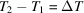. To increase the accuracy of the difference ΔT, this interval should be large. The measured thermal expansionis usually assigned to a temperature at the midpoint of the temperature interval,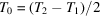. This procedure is only justified if thermal expansion does not depend on temperature.

Since, in fact, thermal expansion depends on temperature, in principle, smaller intervals should be chosen, which, in turn, enlarge the error of ΔT. Here, a compromise has to be made. Sometimes, after completion of a first run and after reviewing the preliminary course of, it is necessary to repeat some measurements using smaller temperature intervals in temperature ranges with large curvatures.

The more-or-less curved course ofis usually fitted by polynomials in powers of temperature. Here, those T terms should be selected that are physically meaningful in the particular temperature range. For the low-temperature behaviour of a metal, a polynomial of typeshould be chosen. For minerals at higher temperatures, a polynomialis used (Saxena & Shen, 1992).

Temperature is usually measured by thermocouples and, in the cases of optical or electrical measurements (Sections 1.4.3.3and 1.4.3.4) and at low temperatures also by platinum resistance thermometers. Above 1100 K, optical pyrometers can be used.

In order to measure the thermal expansion of a crystal, at least as many independent measurements are necessary as the tensor has independent components (fourth column in Table 1.4.1.1). It is advisable, however, to carry out more measurements than are necessary. In this case (of redundancy), a best' set of tensor components is to be determined by least-squares methods as described below.

Let us assume the most general case of a triclinic crystal, where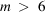independent measurements of thermal expansions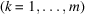were performed along m different directions with direction cosines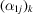with respect to the chosen coordinate system. Each measurementis related to the six unknown tensor components(to be determined) by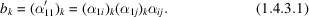If theare replaced by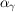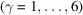, using Voigt's one-index notation (Section 1.1.4.10.2), then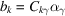represents an overdetermined inhomogeneous system of m linear equations for the six unknowns. The coefficients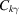, forming anmatrix, are products containing direction cosines according to (1.4.3.1). The solution is obtained after several matrix calculations which are indicated by the formula (Nye, 1985)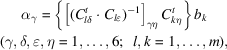where a superscript t' means transposed.

Instead of determining the tensor components of a triclinic or monoclinic crystal in a direct way, as outlined above, it is also possible to determine first the temperature change of the crystallographic unit cell and then, by formulae given e.g. by Schlenker et al. (1978), to deduce the tensor components. The direct approach is recommended, however, for reasons of the propagation of errors (Jessen & Küppers, 1991).

The experimental techniques of measuring relative length changesthat are most widely used include diffraction, optical interferometry, pushrod dilatometry and electrical capacitance methods. If the specimens available are very small and/or irregular in shape, only diffraction methods can be used. The other methods require single-crystal parallelepipedal samples with at least 5 mm side lengths.

#### 1.4.3.2. Diffraction

| top | pdf |

Thermal expansion expresses itself, on a microscopic scale, by a change of the interplanar spacings of lattice planes. These can be measured by use of diffraction methods from changes of Bragg angles. Differentiation of the Bragg equation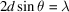, giving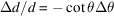, yields the thermal expansions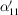in directions normal to lattice planes (hkl) (i.e. along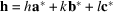) and, if h has direction cosines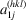with respect to the chosen Cartesian coordinate system,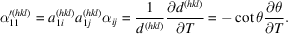The coefficientpermits a tremendous increase of sensitivity and accuracy if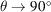. That means, if possible, high-angle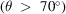reflections should be used for measurement because, for a given Δd, the changes of Bragg angles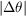to be measured increase with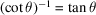.

The most important diffraction techniques (X-radiation is preferentially used) are: the rotating-crystal method, the Weissenberg method and diffractometers with counter recording. If small single crystals (approximately 50 µm) are not available, powder methods (using a Debye–Scherrer film camera or powder diffractometer) must be used, although the advantage of the highly accurate back-reflections, in general, cannot be used.

Experimental aspects of measuring absolute d-values are discussed in detail in Volume C of International Tables for Crystallography (2004), Part 5. Since only relative displacements are to be measured in the present case, many complications connected with the determination of absolute values do not apply for thermal expansion measurements, such as zero-point correction, eccentricity of the mounted sample, refraction, absorption and diffraction profile.

#### 1.4.3.3. Optical methods (interferometry)

| top | pdf |

The basic principle of measuring thermal expansion by interferometry consists of converting sample-length changes into variations of optical path differences of two coherent monochromatic light beams, which are reflected from two opposite end faces of the sample (or planes corresponding to them). An He–Ne laser usually serves as a light source. A beam expander produces a parallel beam and interference by two planes, which are slightly inclined to each other, produces fringes of equal thickness. Thermal expansion causes a movement of this fringe pattern, which is detected by photodiodes. The number of fringes passing a reference mark is counted and gives a measure of the relative movement of the two planes.

As examples for various realizations of interferometric devices (Hahn, 1998), two basic designs will be described.

• (i) Fizeau interferometer (Fig. 1.4.3.1). The sample S is covered by a thin plate(with a polished upper surface and a coarsely ground and non-reflecting lower surface) and is placed in between a bottom plateand a wedge-shaped plate(wedge angle of about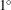). The upper surface ofreflects the incident beam (i) to a reflected beam (r) so that it is removed from the interference process. The relevant interference takes place between ray (1) reflected by the lower surface ofand ray (2) reflected by the upper surface of. A cylindrical tube T, which defines the distance betweenandas well as, is usually made of fused silica, a material of low and well known thermal expansion. The measured dilatation is caused, therefore, by the difference between thermal expansion of the sample and a portion of the fused silica tube of equal length. The whole apparatus is mounted in a thermostat.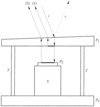Figure 1.4.3.1 | top | pdf |Schematic diagram of a Fizeau interferometer.
• (ii) Michelson interferometer (Fig. 1.4.3.2). The reference mirror M and the beam-splitter B are placed outside the thermostat. The upper face of the sample S is one interference plane and the upper surface of the bottom plate is the other. The interference pattern IP is divided into two fields corresponding to the two ends of the sample. The difference of fringe movements within these two fields yields the absolute thermal expansion of the sample.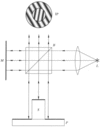Figure 1.4.3.2 | top | pdf |Schematic diagram of a Michelson interferometer.

| top | pdf |

#### 1.4.3.4.1. Inductance changes (pushrod dilatometry)

| top | pdf |

With this method, the expansion of the crystal is transmitted out of the cooled or heated region to an external measuring device by a rod made of a reference material whose thermal expansion is low and well known (usually silica glass) (cf. Gaal, 1998). If this rod is inside a tube of the same material (silica glass), and the specimen is inside as well, then the difference in expansion between the crystal and an equal length of the reference material is measured. Above 1100 K, instead of silica glass, high-purity alumina or single-crystal sapphire or tungsten rods are used.

To measure the displacement of the rods, several techniques are used. The most important are:

 (1) a ferrite core is moved in a coil to change the inductivity of the coil, which is detected by the change of resonance frequency of an electrical circuit having a fixed capacitance; (2) linear-variable-differential transformers.

Temperature gradients in the rod and the tube can lead to severe complications. For every determination, the system should be calibrated by certified materials (White, 1998), such as α-Al2O3, Cu, Pt, fused silica, Si, W, Mg or Mo.

#### 1.4.3.4.2. Capacitance methods

| top | pdf |

In a way similar to the interferometric methods, the change of the gap between the lower surface of P1 and the upper surface of P2 (Fig. 1.4.3.1) is used to determine the thermal expansion of the sample. This gap – with electrically conducting surfaces – is used as the capacitance in an electric circuit with a fixed inductance. The change of capacitance leads to a change of resonance frequency, which is measured.

### References

International Tables for Crystallography (2004). Vol. C. Mathematical, physical and chemical tables, 3rd ed., edited by E. Prince. Dordrecht: Kluwer Academic Publishers.
Gaal, P. S. (1998). Pushrod dilatometers. In Thermal expansion of solids, edited by C. Y. Ho, ch. 5. Materials Park, Ohio: ASM International.
Hahn, T. A. (1998). Thermal expansion measurements using optical interferometry. In Thermal expansion of solids, edited by C. Y. Ho, ch. 6. Materials Park, Ohio: ASM International.
Jessen, S. M. & Küppers, H. (1991). The precision of thermal-expansion tensors of triclinic and monoclinic crystals. J. Appl. Cryst. 24, 239–242.
Nye, J. F. (1985). Physical properties of crystals. Oxford: Clarendon Press.
Saxena, S. K. & Shen, G. (1992). Assessed data on heat capacity, thermal expansion, and compressibility of some oxides and silicates. J. Geophys. Res. 97, 19813–19825.
Schlenker, J. L., Gibbs, G. V. & Boisen, M. B. (1978). Strain-tensor components expressed in terms of lattice parameters. Acta Cryst. A34, 52–54.
White, G. K. (1998). Thermal expansion reference materials. In Thermal expansion of solids, edited by C. Y. Ho, ch. 11. Materials Park, Ohio: ASM International.